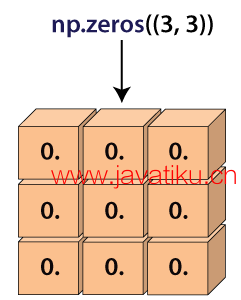# NumPy教程-numpy.zeros() 在 Python 中的使用numpy.zeros() 函数是机器学习程序中广泛使用的最重要的函数之一。该函数用于生成一个包含零的数组。

numpy.zeros() 函数生成一个给定形状和类型的新数组，数组中的元素都填充为零。### 语法

``numpy.zeros(shape, dtype=float, order='C'  ``

### 参数

shape: int 或 int 元组

dtype: 数据类型（可选）

order: {'C', 'F'}（可选）

### 示例 1: 不使用 dtype 和 order 的 numpy.zeros()

``````import numpy as np
a=np.zeros(6)
a  ``````

``array([0., 0., 0., 0., 0., 0.])``

• 我们导入了别名为 np 的 numpy 库。
• 我们声明了变量 'a'，并将 np.zeros() 函数的返回值赋给了它。
• 我们在函数中传递了一个整数值。
• 最后，我们尝试打印变量 'a' 的值。

### 示例 2: 不使用 order 的 numpy.zeros()

``````import numpy as np
a=np.zeros((6,), dtype=int)
a   array([0, 0, 0, 0, 0, 0])``````

``array([0, 0, 0, 0, 0, 0])``

### 示例 3: 使用 shape 的 numpy.zeros()

``````import numpy as np
a=np.zeros((6,), dtype=int)
a   ``````

``````array([[0., 0.],
[0., 0.],
[0., 0.],
[0., 0.],
[0., 0.],
[0., 0.]])``````

• 我们导入了别名为 np 的 numpy 库。
• 我们声明了变量 'a'，并将 np.zeros() 函数的返回值赋给了它。
• 我们在函数中传递了数组元素的形状。
• 最后，我们尝试打印变量 'a' 的值。

### 示例 4: 使用 shape 的 numpy.zeros()

``````Import numpy as np
s1=(3,2)
a=np.zeros(s1)
a  ``````

``````array([[0., 0.],
[0., 0.],
[0., 0.]])``````

### 示例 5: 使用自定义 dtype 的 numpy.zeros()

``````Import numpy as np
a=np.zeros((3,), dtype=[('x', 'i4'), ('y', 'i4')])
a  ``````

``array([(0, 0), (0, 0), (0, 0)], dtype=[('x', '<i4'), ('y', '<i4')])``

• 我们导入了别名为 np 的 numpy 库。
• 我们声明了变量 'a'，并将 np.zeros() 函数的返回值赋给了它。
• 我们在函数中传递了数组元素的形状和自定义数据类型。
• 最后，我们尝试打印变量 'a' 的值。